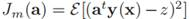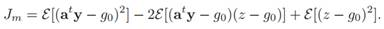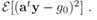# Consider the criterion function  and the Bayes discriminant function g0(x). (a) Show that (b) Use the fact that the conditional mean of z is g0(x) in showing that the aˆ that minimizes Jm also minimizes

Question
1 views

Consider the criterion functionand the Bayes discriminant function g0(x).

(a) Show that(b) Use the fact that the conditional mean of z is g0(x) in showing that the aˆ that minimizes Jm also minimizes### This question hasn't been answered yet.